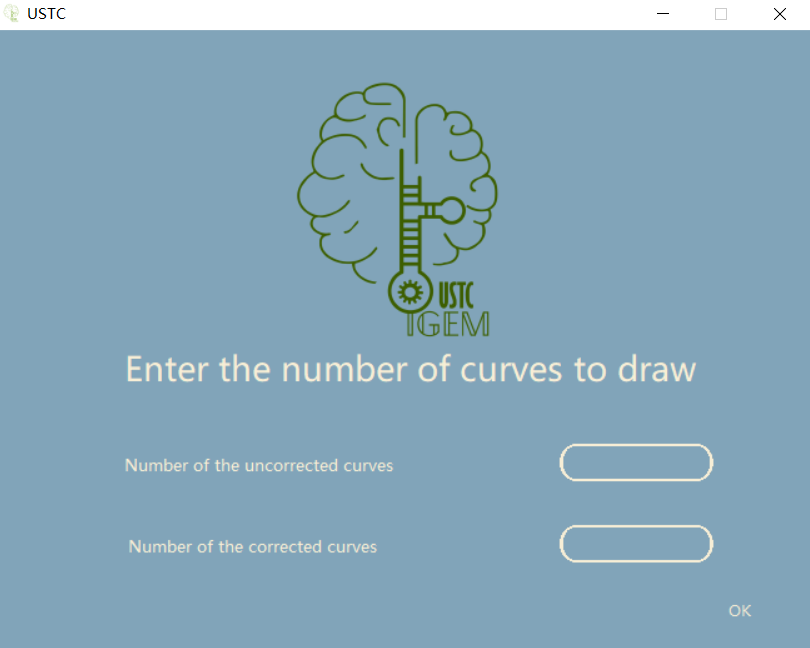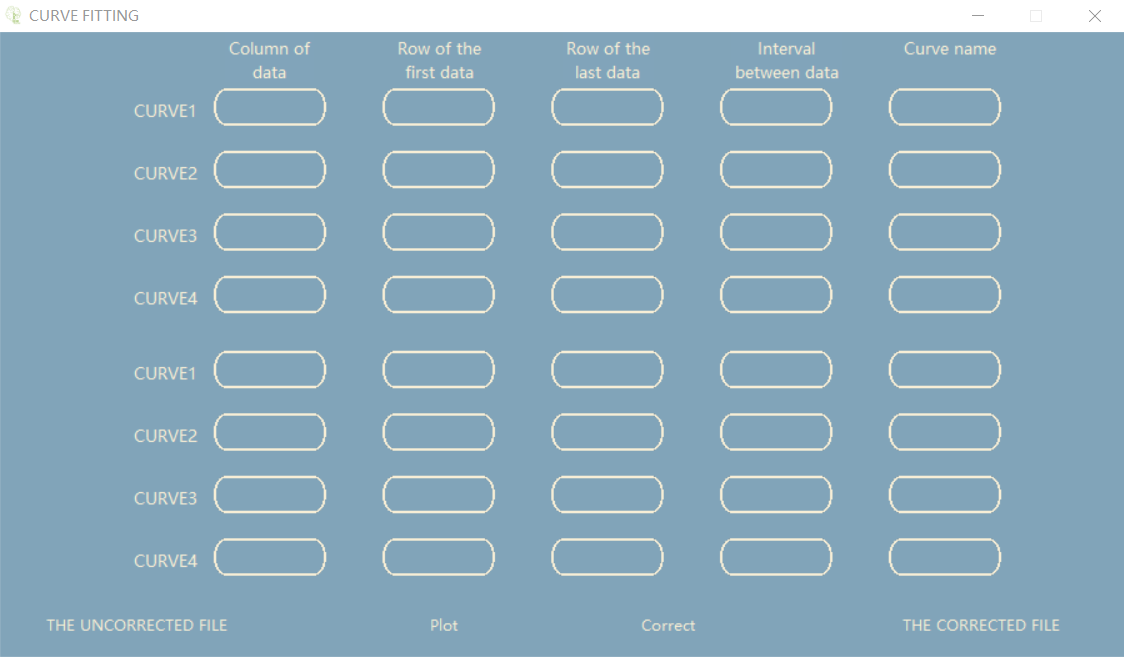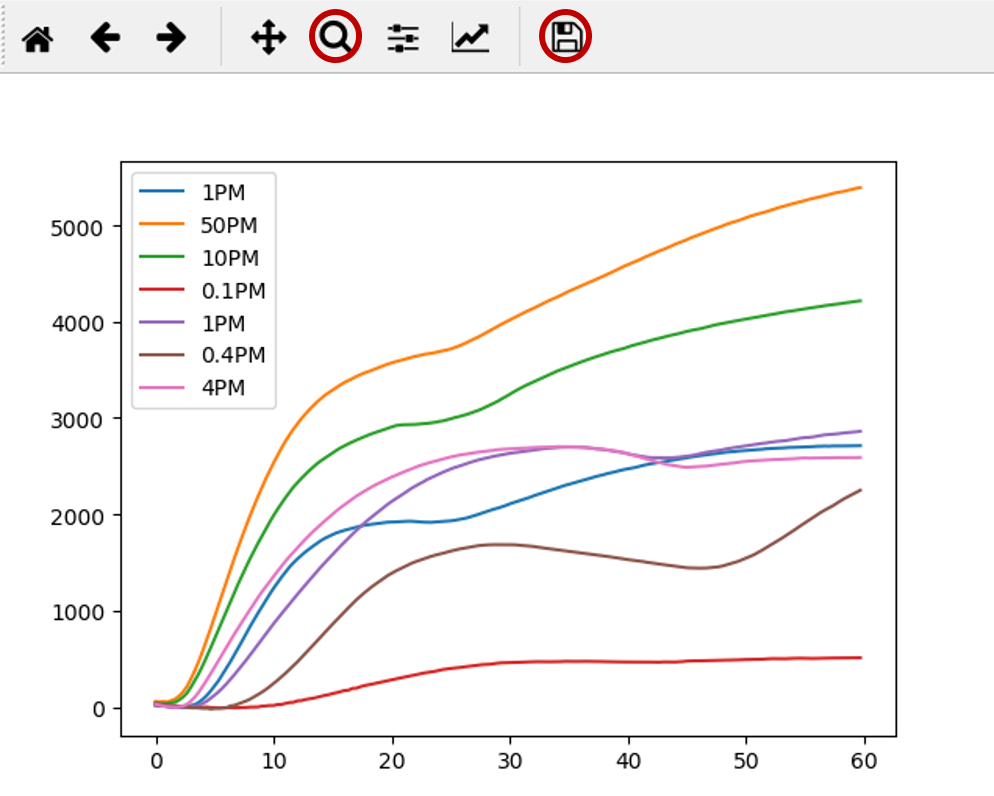# Team:USTC/Software

USTC-iGEM-software

# Software Development Background

In the actual control experiment of each group, the relationship between fluorescence intensity and time is different even if the template with the same concentration is in each group of reaction because the reaction start time is different from the measurement start time in the experiment and the instrument is also affected by uncertain factors. However, according to the experimental image, it can be found that the error influence mentioned above does not change the trend of the experimental image, but only changes the size of the specific value in the image. Therefore, our team redesigned the experimental scheme according to this experimental phenomenon, and designed a software to correct the changes in experimental images caused by such errors.

# Software Instructions

1. When opening this program, users can see the initial interface as shown behind.

2. In the “Number of the uncorrected curves” line, enter the number of curves(0~4). These curves are from the same set of experiments and are used as standard curves in the correcting function.

3. In the "Number of the corrected curves" line, enter the number of corrected curves (0 ~ 4).These curves are from the same set of experiments and are used as the curves to be corrected in the correcting function.4. After entering the numbers, click "OK" button to enter the next interface

5. The interface is divided into three parts.

6. Part 1 is the uncorrected data input box, which is divided into CURVE1, CURVE2...Line. The number of lines here is the value entered in the first line of the previous interface.The first line in the data input box here serves as the correcting curve data location.The first input box inputs the number of columns of the file data (data read only supports in a row). The second input box inputs the line number of the first data. The third input box inputs the line number of the last data.The fourth input box inputs the interval between data. The fifth input box inputs the label of the curve.The following input boxes all enter data in this way.

7. Part 2 is the corrected data input box, which is divided into CURVE1, CURVE2...Line. The number of lines here is the value entered in the first line of the previous interface.The first line in the data input box here serves as the correcting curve data location(The first row of the two parts in the experiment requires data from the experimental group at the same concentration).

8. Part 3 has four buttons.Button 1 is named “THE UNCORRECTED FILE”.You can select a file with the XLS suffix for storing data by pushing this button. The data in this file is selected as the data that will not be corrected in the correcting function.

9. Button 2 is named “PLOT”.You can graph the input data by pushing this button. This function only provides drawing function and does not provide correction function.

10. Button 3 is named “Correct”. You can correcting the data and then graph the correcting data by pushing this button.This function provides correcting function.

11. Button 4 is named “THE CORRECTED FILE”. You can select a file with the XLS suffix for storing data by pushing this button. The data in this file is selected as the data that will be corrected in the correcting function.12. You can get something like the image beneath by pushing the "Plot" or "Correct" button after you have entered the data.The function key marked with red circle on the left can be used to enlarge the image, and the function marked with red circle on the right can be used to save the image.# Attention

1. In order to improve the operation speed of the correction function, the software limits the correction curve and only supports the contractile correction of the corrected curve, that is, left and down correction.

2. This software only supports reading files with the suffix XLS.

3. This software is designed to address the current experimental results. In fact there are some alternatives, such as adding standard sample sets to the same batch before each measurement is performed and plotting the standard curve. But this method is obviously not convenient enough, so we propose the design of the software.

4. In the future, we plan to combine software and hardware to input current signals, convert them into curves, output and store target protein concentrations. We will also make the graphical interface more aesthetically pleasing and easier to use for users.